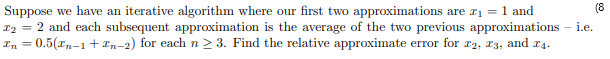# we have an iterative algorithm where our first two approximations are r = 1 andSuppose2 and each subsequent approximation is the average of the two previous approximationsEn0.5(r-+xn-2) for each n 2 3. Find the relative approximate error for r2, T3, and r4i.eI2

Question
6 viewshelp_outlineImage Transcriptionclosewe have an iterative algorithm where our first two approximations are r = 1 and Suppose 2 and each subsequent approximation is the average of the two previous approximations En0.5(r-+xn-2) for each n 2 3. Find the relative approximate error for r2, T3, and r4 i.e I2 fullscreen
check_circle

Step 1

The values of x1 and x2 are 1 and 2, respectively. And the values of the x3...

### Want to see the full answer?

See Solution

#### Want to see this answer and more?

Solutions are written by subject experts who are available 24/7. Questions are typically answered within 1 hour.*

See Solution
*Response times may vary by subject and question.
Tagged in

### Other